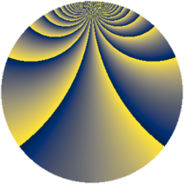# Properties

 Label 770.2.brLevel $770$ Weight $2$ Character orbit 770.br Rep. character $\chi_{770}(19,\cdot)$ Character field $\Q(\zeta_{30})$ Dimension $384$ Newform subspaces $2$ Sturm bound $288$ Trace bound $2$

# Related objects

## Defining parameters

 Level: $$N$$ $$=$$ $$770 = 2 \cdot 5 \cdot 7 \cdot 11$$ Weight: $$k$$ $$=$$ $$2$$ Character orbit: $$[\chi]$$ $$=$$ 770.br (of order $$30$$ and degree $$8$$) Character conductor: $$\operatorname{cond}(\chi)$$ $$=$$ $$385$$ Character field: $$\Q(\zeta_{30})$$ Newform subspaces: $$2$$ Sturm bound: $$288$$ Trace bound: $$2$$ Distinguishing $$T_p$$: $$3$$

## Dimensions

The following table gives the dimensions of various subspaces of $$M_{2}(770, [\chi])$$.

Total New Old
Modular forms 1216 384 832
Cusp forms 1088 384 704
Eisenstein series 128 0 128

## Trace form

 $$384q + 48q^{4} + 12q^{5} + 36q^{9} + O(q^{10})$$ $$384q + 48q^{4} + 12q^{5} + 36q^{9} - 2q^{11} + 6q^{14} + 12q^{15} + 48q^{16} - 20q^{25} + 12q^{26} + 36q^{31} - 30q^{35} - 112q^{36} - 20q^{39} + 30q^{40} - 22q^{44} - 12q^{45} - 8q^{49} - 60q^{51} + 8q^{56} + 4q^{60} - 60q^{61} - 96q^{64} - 192q^{66} + 54q^{70} - 80q^{71} + 60q^{75} + 18q^{80} - 36q^{81} + 20q^{85} - 36q^{86} - 120q^{89} + 2q^{91} + 150q^{94} + 90q^{95} - 44q^{99} + O(q^{100})$$

## Decomposition of $$S_{2}^{\mathrm{new}}(770, [\chi])$$ into newform subspaces

Label Dim. $$A$$ Field CM Traces $q$-expansion
$$a_2$$ $$a_3$$ $$a_5$$ $$a_7$$
770.2.br.a $$192$$ $$6.148$$ None $$-24$$ $$0$$ $$6$$ $$6$$
770.2.br.b $$192$$ $$6.148$$ None $$24$$ $$0$$ $$6$$ $$-6$$

## Decomposition of $$S_{2}^{\mathrm{old}}(770, [\chi])$$ into lower level spaces

$$S_{2}^{\mathrm{old}}(770, [\chi]) \cong$$ $$S_{2}^{\mathrm{new}}(385, [\chi])$$$$^{\oplus 2}$$